# How to code a homing attack? (Read 1398 times)

Started by QuickFist, June 26, 2017, 06:24:48 pm

#### QuickFist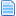##### How to code a homing attack?
#1  June 26, 2017, 06:24:48 pm
Just as the title says, I'm trying to code a homing attack but I don't know how to code the vels to work like that.
Also, I'm having issues with the change state, or with the flight state, I'm not sure what's wrong.
;---------------------------------------------------------
; Homing Attack Start - from ground
[statedef 950]
type    = a
physics = a
anim = 950

[state 50, 6]
type = turn
trigger1 = p2dist x < 0

[State 1155, VelSet]
type = VelSet
trigger1 = time = 0
y = -7

trigger1 = animelem = 5
y = .75

trigger1 = animelem = 6
y = 0

[state 50, 12]
type = changestate
trigger1 = anim=950
trigger1 = animelem = 7
value = 951
;---------------------------------------------------------
; Homing Attack flight
[statedef 951]
type    = a
physics = a
anim = 951

[state 50, 6]
type = turn
trigger1 = p2dist x < 0

[state 50, 7]
type = velset
trigger1 = Time = 0
x = 8

[State 1200, Hitdef]
type      = HitDef
trigger1      = !Time
attr      = A, SA
hitflag      = MAF
guardflag      = MA
;animtype = hard
priority      = 7, Hit
damage      = 0
;Ceil(IfElse(fvar(11) * (20+3*(var(5)=1)+6*(var(5)=2)) < 7, 7, fvar(11) * (20+3*(var(5)=1)+6*(var(5)=2)))), 2+(var(5)=1)+2*(var(5)=2)
;20+3*(var(5)=1)+6*(var(5)=2),2+(var(5)=1)+2*(var(5)=2)
pausetime      = 1,1
sparkno      = -1
guard.sparkno   = -1
sparkxy      = -10, -9
hitsound      = -1
guardsound   = -1
p2facing      = 1
ground.type   = low
ground.slidetime   = 12
ground.hittime   = 12
ground.velocity   = -2,-9
air.velocity   = -2,-8
;fall = 1
fall.recover   = 0
getpower      = IfElse(P2MoveType = H,40,140), 35
givepower      = 35,35
envshake.time   = 16
envshake.freq   = 60
envshake.ampl   = 4
yaccel      = 0.5
;p1stateno = 1202
persistent   = 0
;snap      = 20,50

[State 1200, TargetBind]
type = TargetBind
trigger1 = MoveHit = 1
time = 1
pos = 5+p2bodydist x, p2bodydist y;60
ignorehitpause = 1
persistent = 0

[State 1200, ChangeState]
type = ChangeState
trigger1 = MoveHit = 1 && Enemy,Movetype = H ;if you write "Target, MoveType = H" you'll get debug flood.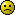value = 952
ctrl = 0
ignorehitpause = 1
persistent = 0

;---------------------------------------------------------
; Homing Attack hit
[statedef 952]
type    = A
movetype = A
physics = A
Anim = 610
;velset = 0,0
sprpriority = 2
ctrl = 0

[State 610, 1]
type = playsnd
trigger1 = animelem = 1 && random <= 800
value = s2000,0+random%4
volume = 255
channel = 0

[State 610, 2]
type = Playsnd
trigger1 = animelem = 1
value = s4000,1

[State 610, 3]
type = HitDef
trigger1 = Time = 0
attr = A, NA
damage = 22
animtype = Medium
hitflag = MAF
guardflag = HA
pausetime = 14,14
sparkxy = 0,-40
sparkno = s9104
guard.sparkno = s9101
hitsound = s5544,1
guardsound = s5546,0
ground.type = low
air.type = High
ground.slidetime = 12
ground.hittime = 12
air.hittime = 12
guard.ctrltime = 15
ground.velocity = -1
guard.velocity = -5
air.velocity = -1,-7.25
airguard.velocity = -5,-1
ground.cornerpush.veloff = 0
air.cornerpush.veloff = 0
airguard.cornerpush.veloff = 0
getpower = 35,35
givepower = 35, 35
yaccel = .5
fall.xvelocity =0
fall.yvelocity =-2

[State 600, Changestate]
type = ChangeState
trigger1 = AnimTime = 0
value = 460

The char jumps and it seemingly changes to the flight state but It lands inmediately. What I want it to do: jump, stay in the air for like 20 ticks, then change to the flight, follow P2 vertically and when in contact, hit.Last Edit: June 26, 2017, 07:30:27 pm by QuickFist

#### Noctis##### Re: How to code a homing attack?
#2  June 26, 2017, 11:00:15 pm
So you don't want an actual homing attack, you just want to match your opponents vertical velocity? Or fly to his location vertically? Not horizontally?
Just following your opponent vertically should be as simple as something like..

[state 0, velset]
type=velset
trigger1=1
y=enemynear,vel y
ignorehitpause=1

somethin' like that.

#### QuickFist##### Re: How to code a homing attack?
#3  June 26, 2017, 11:06:04 pm
That works for the Y vel, but I still need it to fly towards p2.
Look, what I'm aiming for is the DBZ pursuit attack#### Odb718##### Re: How to code a homing attack?
#4  June 27, 2017, 04:48:55 am
••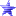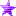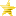• Shame on you!

[state 50, 12]
type = changestate
trigger1 = anim=950  ;redundant. There's no way for it not to be 950 from what I can tell
trigger1 = animelem = 7 ;change this to >=7 to avoid problems
value = 951

[state 50, 6]
type = turn
trigger1 = p2dist x < 0  ;having it at 0 will SNAP the player. It may cause jitters if P2 is hovering above P1.

[state 50, 7]
type = velset
trigger1 = Time = 0
x = 8
So where's the velset/veladd for when P2 moves?

I'm not familiar with the DBZ move. But you're going to want to slow down the vel x to switch which way it's heading.
I'd say look at Shadow Lady's homing missiles. They're pretty relentless. My Samus' homing missiles dont bother going backwards.